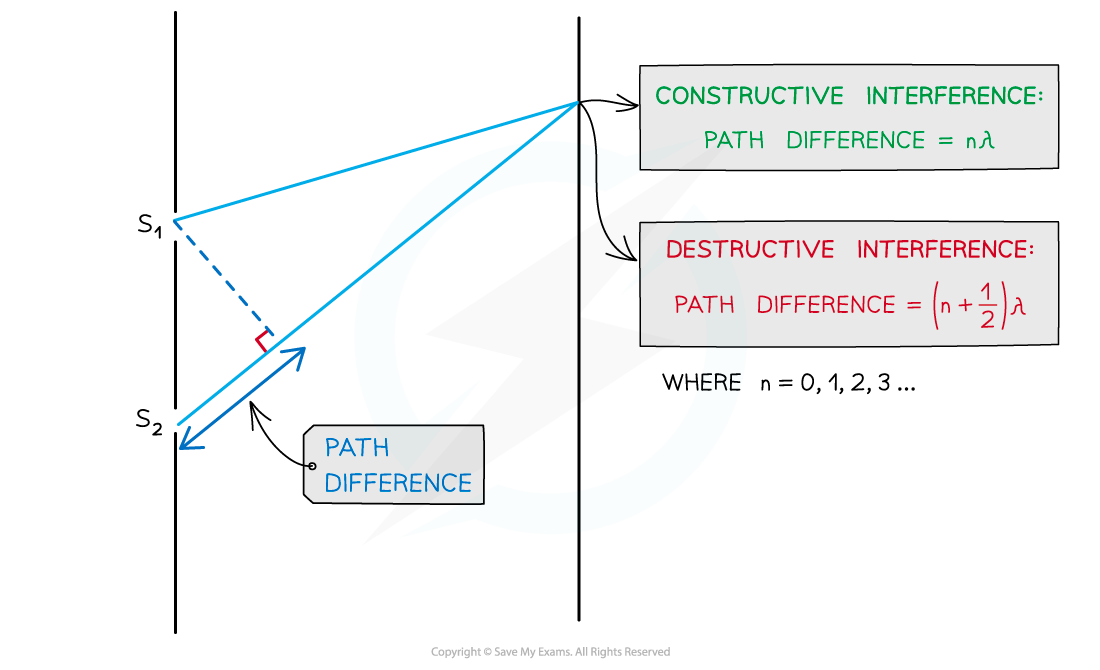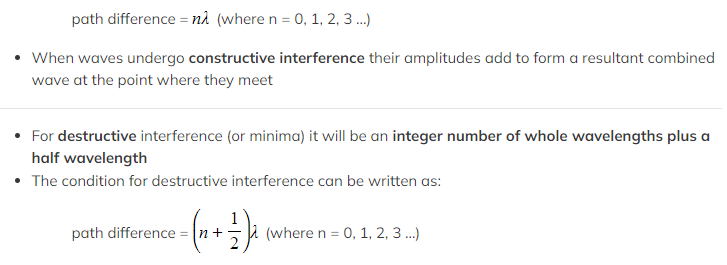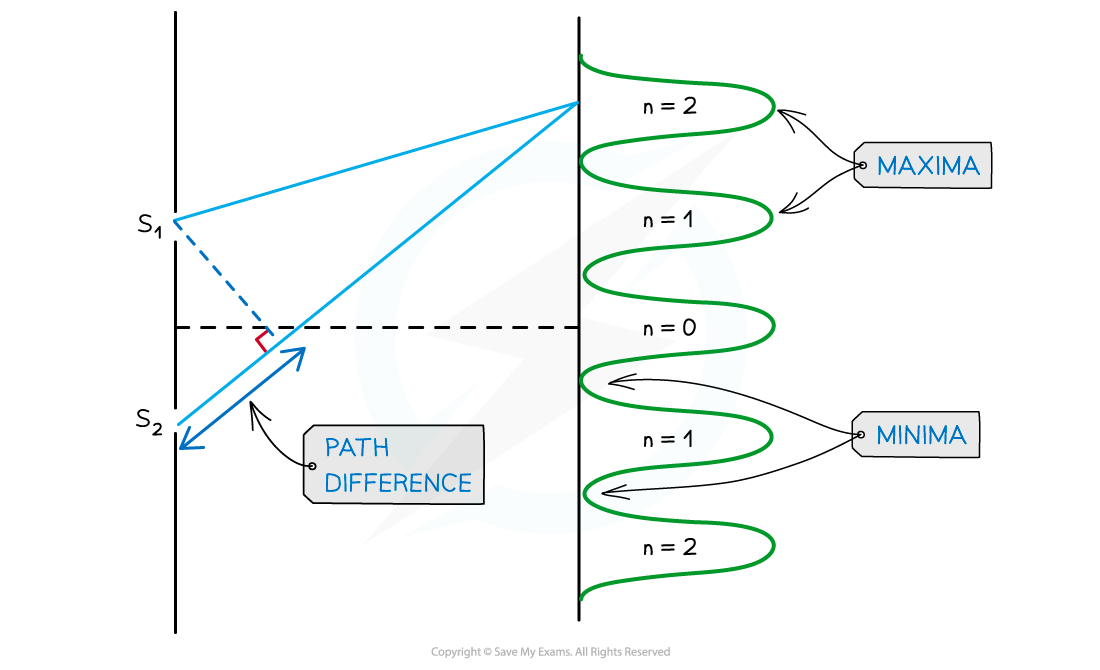# IB DP Physics: HL复习笔记9.3.2 Two-Slit Interference Patterns

### Two-Slit Interference Patterns

• For two-source interference fringes to be observed, the sources of the wave must be:
• Coherent (constant phase difference)
• Monochromatic (single wavelength)
• When two waves interfere, the resultant wave depends on the phase difference between the two waves
• This is proportional to the path difference between the waves which can be written in terms of the wavelength λ of the wave
• As seen from the diagram, the wave from slit S2 has to travel slightly further than that from S1 to reach the same point on the screen
• The difference in this distance is the path differenceInterference is caused by the variation in path length between the two slits

• For constructive interference (or maxima), the difference in wavelengths will be an integer number of whole wavelengths
• The condition for constructive interference can be written as:• n is the order of the maxima (bright fringes) / minima (dark fringes)
• n = 0 is taken from the middle, n = 1 is the next peak and so on
• When waves undergo destructive interference their amplitudes subtract to form a resultant combined wave at the point where they meet
• The equations above are the same versions as found in your data booklet
• Don't let other versions of these equations confuse you
• Constructive and destructive interference can be calculated with alternative equations for specific values of nInterference pattern of light waves shown with orders of maxima and minima

• An interference pattern shows the intensity of light at different distances away from the central maxima
• For a double-slit interference pattern the intensity of the light is the same for all maxima
• This is different to the double-slit diffraction pattern that is observed on a screen (These are explained in 9.3.3 Diffraction Grating Patterns)

#### Double-Slit Diffraction Geometry

• The diffraction pattern made by waves passing through two slits of separation d can be observed on a screen placed a large distance, D, away• If the distance, D, between the slits and the screen is considerably larger than the slit separation, [Image_Link]data:;base64,